# Multiple Strata in dssd

Designing distance sampling surveys containing more than one stratum.

Laura Marshall http://distancesampling.org (CREEM, Univ of St Andrews)https://creem.st-andrews.ac.uk
2020-02-02

## Introduction

This vignette assumes the reader is familiar with the topics covered in the getting started vignette. It expands on that content, demonstrating how to use the distance sampling survey design package, dssd (Marshall, 2019) when your study region is made up of multiple strata. This vignette will detail how you can select different designs (within the same design category, either lines or points) for each stratum and provide stratum specific design parameters. Please note that the examples provided in this vignette are designed to make the reader aware of what is possible inside the dssd package and the designs are not necessarily something that we would recommended for these example survey regions.

## Stratified Designs - why stratify?

There are a number of reasons that we may wish to create a stratified design. Firstly, it may be for efficiency reasons. For example, we may wish to divide our region into a number of more convex shapes when using an equal spaced zigzag design to reduce off-effort transit time at the survey region boundary. Figure 1 presents an example of a Minke whale survey, in the left panel when the zigzag design is generated in the study region as a whole we can see that it is fairly inefficient with large distances between the ends of transects (note that only the lengths of transect inside the shaded study area will be surveyed). In the right panel we have reduced the off effort transit time by dividing the study area into a number of strata. As the purpose of this stratification is simply to improve efficiency we would still want to try to achieve equal coverage across all strata.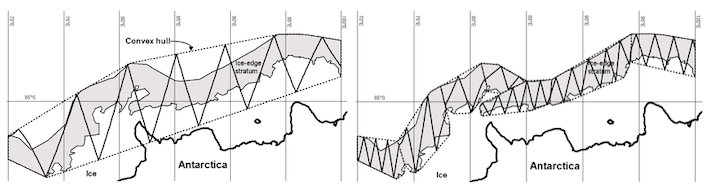Figure 1: Example survey design for a Minke whale survey. The shaded polygons represent the study area and the dotted lines represent convex hulls stretched around the study region polygons. While the full length of the zigzag transects is shown only the parts inside the shaded area will be surveyed. Left panel shows an equal spaced zigzag design generated inside a convex hull applied to the study region as a whole. The right panel shows an equal spaced zigzag design generated inside a number of strata selected so that the study region was divided into a number of almost convex shapes.

Another reason some people consider stratifying their survey region is to improve precision. More precise estimates of density / abundance can sometimes be achieved by allocating more effort to areas of high density and less effort to areas of low density. This allows inter-stratum differences to be estimated rather than have them contribute to the variance of the estimates. Certainly, if there are large areas where you expect to see very little it might be unwise to spend a lot of expensive resources in these areas. However, there are dangers of unequal effort allocation (otherwise called non-uniform coverage). There may be differences in encounter rate, detection function or mean cluster size across the different strata. If any of these are estimated by pooling across strata when in fact they differ between strata then within stratum estimates will be biased and the global estimates may also be biased. The only way to be sure if inter-stratum differences exist is to ensure that you have sufficient data in each strata to estimate the encounter rate, detection function and mean cluster size by stratum. Otherwise you will need to rely on making biological assumptions.

A third scenario in which you may want to stratify is that you wish to make inference about a particular sub-region of your study area. In addition, if you plan on fitting a spatial model to your distance sampling data you may have regions of particular interest in which you would like to detect changes in density at smaller scales than in other regions.

Finally you may wish to stratify as you plan to survey from two different platforms, for example marine surveys when some strata will be surveyed from a ship while others are surveyed from the air. Ship-board surveys often use zigzag designs for efficiency to maximise on-effort survey time. Ships have sufficient space for multiple sets of observers so ship board surveys can achieve continuous observation while travelling along the transects. In contrast, aerial surveys often use systematic parallel line designs. Firstly the tight turns at the end of zigzags can be hard to achieve in an airplane (and unpleasant for the crew) and secondly the time taken to transit between parallel transects can be useful periods of rest for a single set of observers. The Small Cetaceans in the European Atlantic and North Sea II (SCANS II) stata were designed to implement this mixed platform approach.

## Multi-Strata Designs using dssd

### Equal Effort Designs

This survey design package assists with equal effort allocation across strata by allowing input of a single value for either the number of samplers or the length of the line to be shared out equally among strata. When the design is systematic and the same design is used in every strata then dssd can calculate the spacing value across all strata at once and can ensure more even coverage. Alternatively, users can imput a single spacing value to be used in all strata. These strategies lead to as equal effort as possible across the study region. There may be small differences between strata depending on the design selected and the exact shapes of the different strata. When designs vary across strata or are not systematic then the spacing values or sampler numbers must be calculated on a stratum by stratum basis and may lead to slightly larger differences in coverage between strata, such differences can be assessed inside dssd by running coverage simulations. It is generally advisable to run coverage simulations for all potential designs.

### Unequal Effort Designs

If you wish to implement an unequal effort design, then this can be achieved in a number of ways inside dssd. The user can chose to explicitly specify the number of samplers, line length or other design parameter values for each stratum individually. Alternatively, the user can specify a single value for number of samplers or line length and allocate the effort to each stratum based on proportions. If the effort allocation argument is not supplied then the default is that effort is allocated based on stratum area giving approximately equal coverage between strata. Alternatively, the user can instead specify the effort allocation argument as a vector of proportions with one value per stratum which sum to 1. Effort will then be allocated to strata based on these proportions.

## Defining the Study Area

Load the dssd library.


library(dssd)

The study area can be defined in the same way as for a single stratum study region by providing a shapefile. This shapefile however will contain multiple polygon features. The first example study region used in this vignette is one of the Danish coastal strata from the SCANS II survey, Figure 2. The coastline is the study region border along the east of the study area. The study area had been divided into two strata for the purposes of maximising samplers and keeping the samplers roughly perpendicular to the coastline. For the design examples in this vignette we will treat this strata as the entire study region and the parts as separate strata. Note that the units for this shapefile are in metres so all design arguments relating to distances must also be provided in metres.


shapefile.name <- system.file("extdata", "Strata.shp", package = "dssd")
region <- make.region(region.name = "study.area",
strata.name = c("North", "South"),
shape = shapefile.name)
plot(region)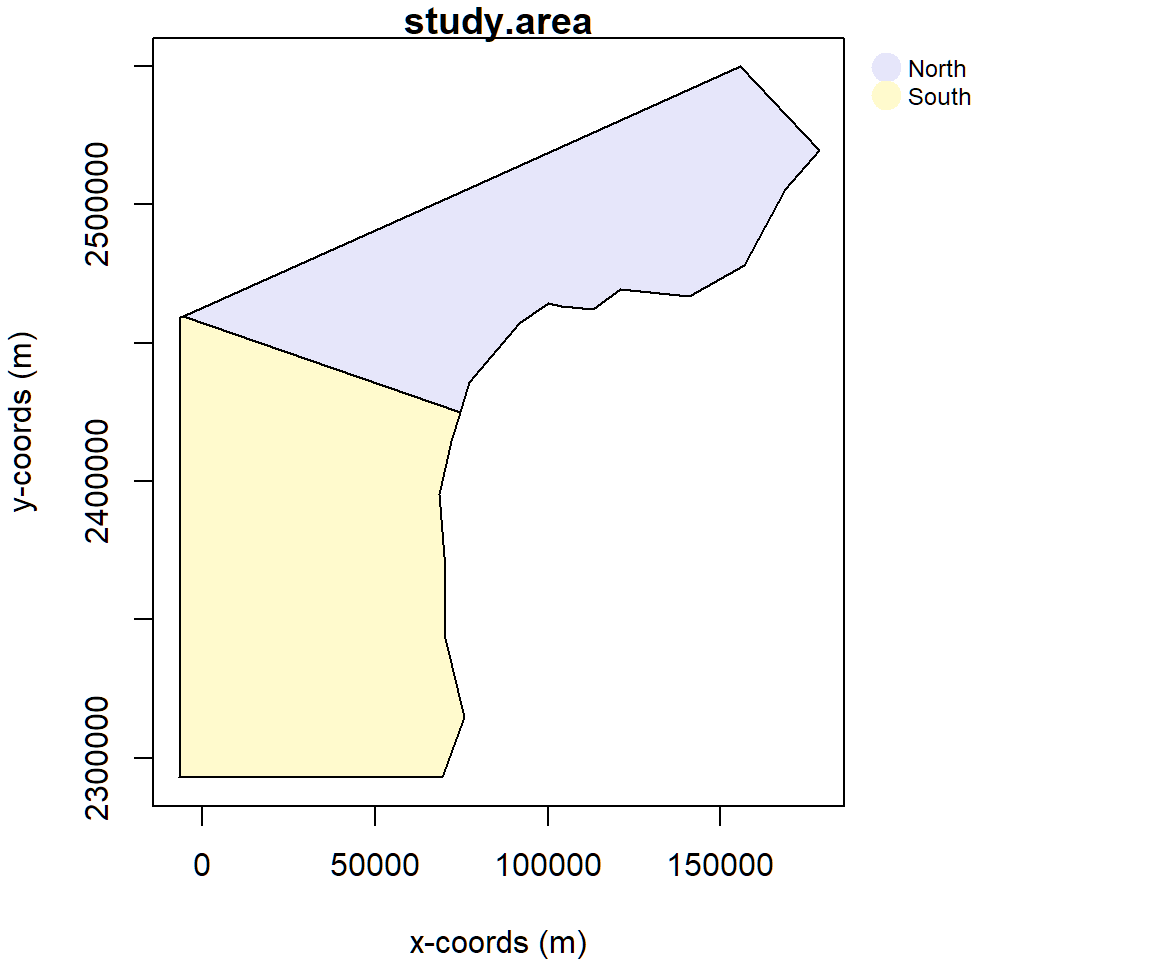Figure 2: Plot of an example study area comprising of 2 strata, a northern stratum and a southern stratum

Additional information on how to manually create multi-strata study regions inside R can be found in the manual creation of study areas appendix.

## Creating a Coverage Grid

The coverage grid can be created in the same way as for a single stratum study region. The creation of the coverage grid involves creating a single grid of points over the entire study area and so is unaffected by the region being subdivided into multiple strata, Figure 3.


cover <- make.coverage(region,
n.grid.points = 1000)
plot(region, cover)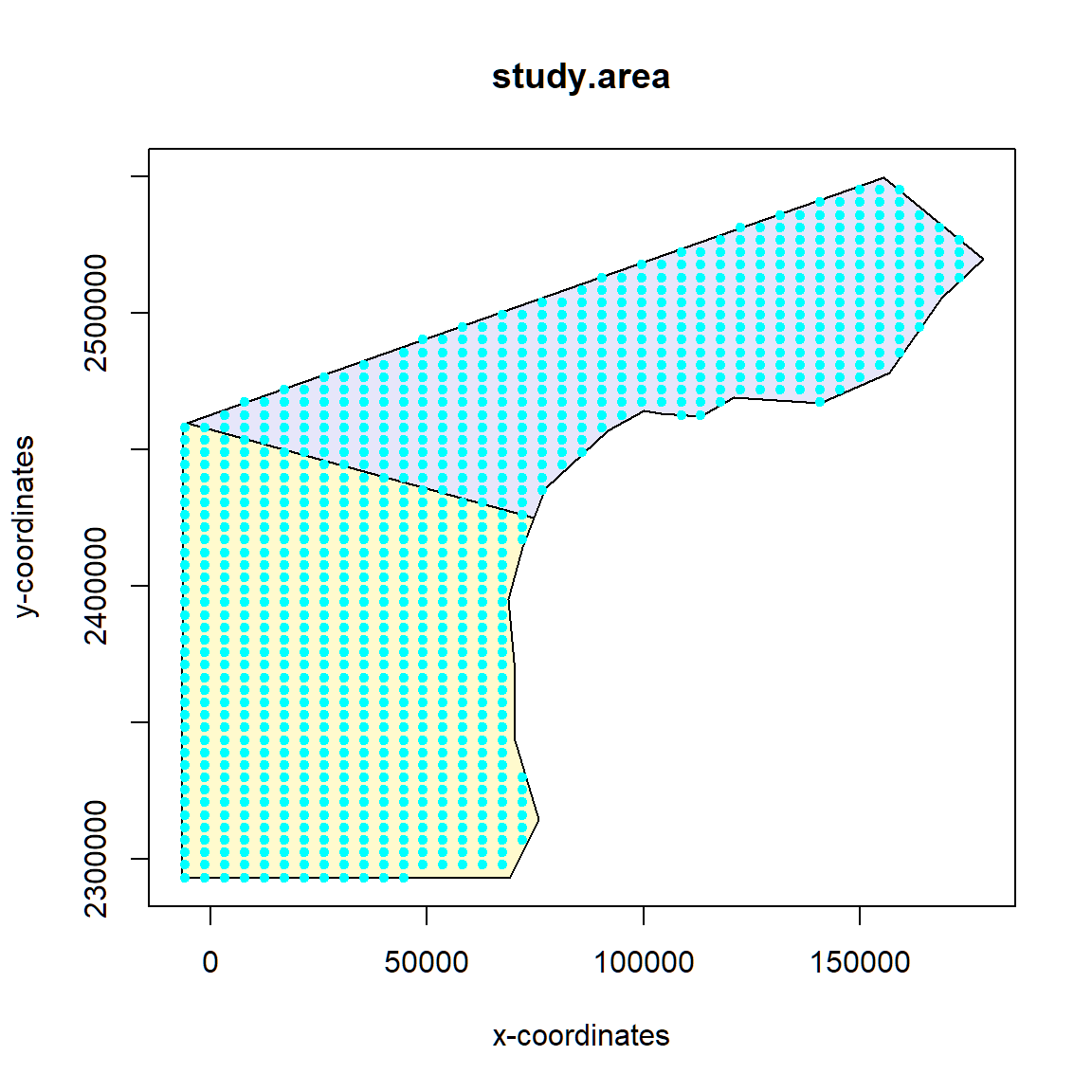Figure 3: Coverage grid with approximately 1000 grid points.

## Defining the Design

This section will demonstrate a number of example designs to show how design, design angle, effort, edge sampling and other design parameters can be varied across strata.

### Default Design (with specified design angles)

Similar to single stratum regions, the default design for multi-strata regions is 20 systematic parallel lines with a design angle of 0 (i.e. the lines run perpendicular to the x-axis) and using a minus sampling edge protocol. The spacing is selected at the global level to achieve a total of approximately 20 lines across all strata. In this first example, we will leave the default effort but specify design angles which will mean that our parallel lines run roughly perpendicular to the coastline. Our survey should therefore have around 20 lines which have the same spacing in both the North and South strata. The default design has a truncation distance of 1 which will rarely be applicable so we have specified it here as 2000 m. A single survey generated from this design is plotted in Figure 4.


default.design <- make.design(region = region,
transect.type = "line",
design = "systematic",
samplers = 20,
design.angle = c(155, 90),
edge.protocol = "minus",
truncation = 2000,
coverage.grid = cover)
transects <- generate.transects(default.design)
plot(region, transects, lwd = 1.5, col = 4)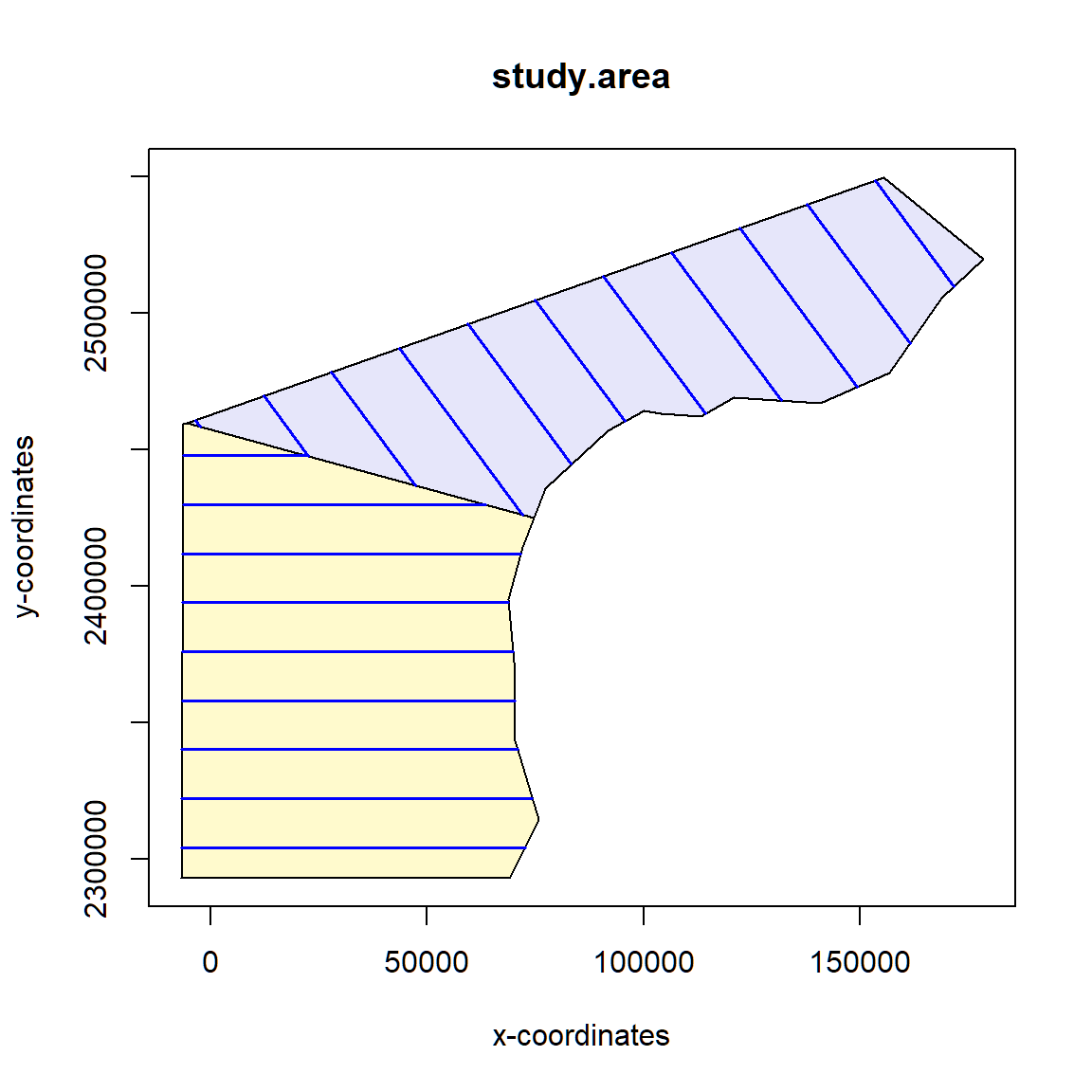Figure 4: Survey generated from on a multi-strata default design with stratum specific design angles.

We can also view a summary of the survey shown in Figure 4. The output shown below indicates that dssd selected a spacing of around 17.9 km (17946.09 m) between the systematic parallel lines. As both strata used the same systematic design the spacing values were calculated globally and are identical for both strata. It also tells us that the percentage of the study region covered was around 22% in both strata and that for this particular survey we have achieved exactly 20 transects, 11 in the northern strata and 9 in the southern strata.


transects

Strata North:
______________
Design:  systematically spaced parallel transects
Spacing:  17946.09
Line length: 525341
Trackline length: 724166.8
Cyclic trackline length: 904116.6
Number of samplers:  11
Design angle:  155
Edge protocol:  minus
Covered area:  2083756793
Strata coverage: 22.45%
Strata area:  9280648398

Strata South:
______________
Design:  systematically spaced parallel transects
Spacing:  17946.09
Line length: 643553.8
Trackline length: 814796.6
Cyclic trackline length: 958365.9
Number of samplers:  9
Design angle:  90
Edge protocol:  minus
Covered area:  2563013876
Strata coverage: 21.88%
Strata area:  11716012130

Study Area Totals:
_________________
Line length: 1168895
Trackline length: 1538963
Cyclic trackline length: 1862483
Number of samplers:  20
Covered area:  4646770669
Average coverage: 22.13%

### Vary design by stratum

Within the categories of line and point transect designs, different types of design may be selected for each stratum. Here we will use an equally spaced zigzag design in the northern stratum and a systematic parallel line in the southern stratum. Note the design angle has a different definition for zigzag designs than parallel line designs so the first value has been modified to 65 degrees (at right angles to the parallel line design above). To maximise the efficiency of the zigzag design we will specify the bounding shape for this stratum as a convex hull. Note that no bounding shape is used in the systematic parallel line design so the second value for bounding shape argument is NA. This design also explicitly declares the edge protocol as minus sampling, which will be applied to both strata. The effort for this design is supplied as a single line length and as we have not defined effort allocation the total line length of 1200 km (1200000 m) will be shared among the strata based on their areas. An example survey generated from this design is shown in Figure 5.


design <- make.design(region = region,
transect.type = "line",
design = c("eszigzag", "systematic"),
line.length = 1200000,
design.angle = c(65, 90),
bounding.shape = c("convex.hull", NA),
edge.protocol = "minus",
truncation = 2000,
coverage.grid = cover)
transects <- generate.transects(design)
plot(region, transects, lwd = 1.5, col = 4)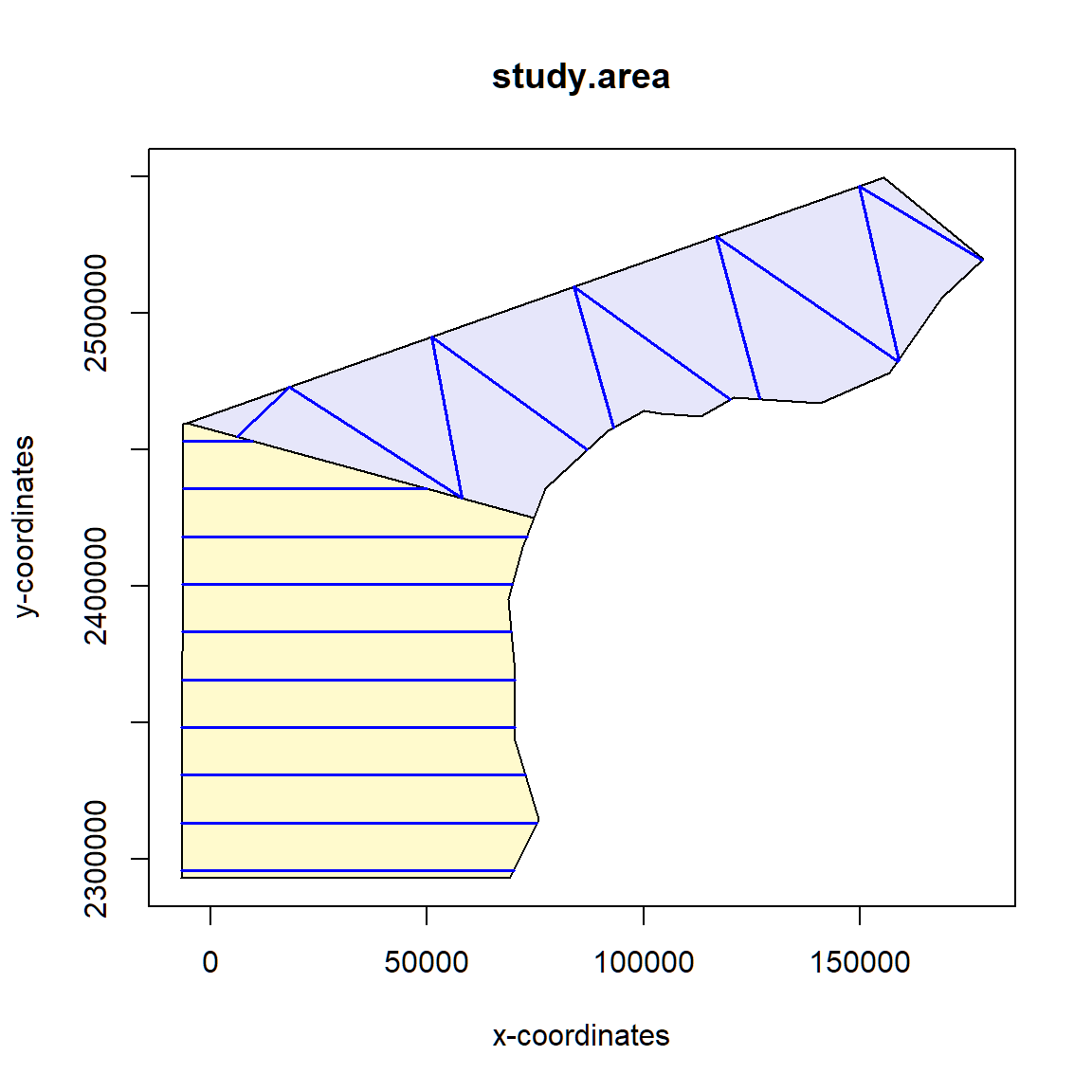Figure 5: Example survey from a mixed-type design with systematic parallel lines in the southern strata and an equal spaced zigzag designs in the northern strata.

Let us now run a coverage check on this design to see if the coverage is roughly the same in both strata. Looking at Figure 6 the coverage probabilities look to be roughly the same on average for the two strata. We do however see the sharp corners of the northern stratum displaying a higher coverage due to the zigzag design being generated in a convex hull.


# You can re-run the coverage simulation using the following code. Note, your
# results should vary slightly from mine, make sure you haven't set a seed!
design <- run.coverage(design, reps = 1000)
plot(design)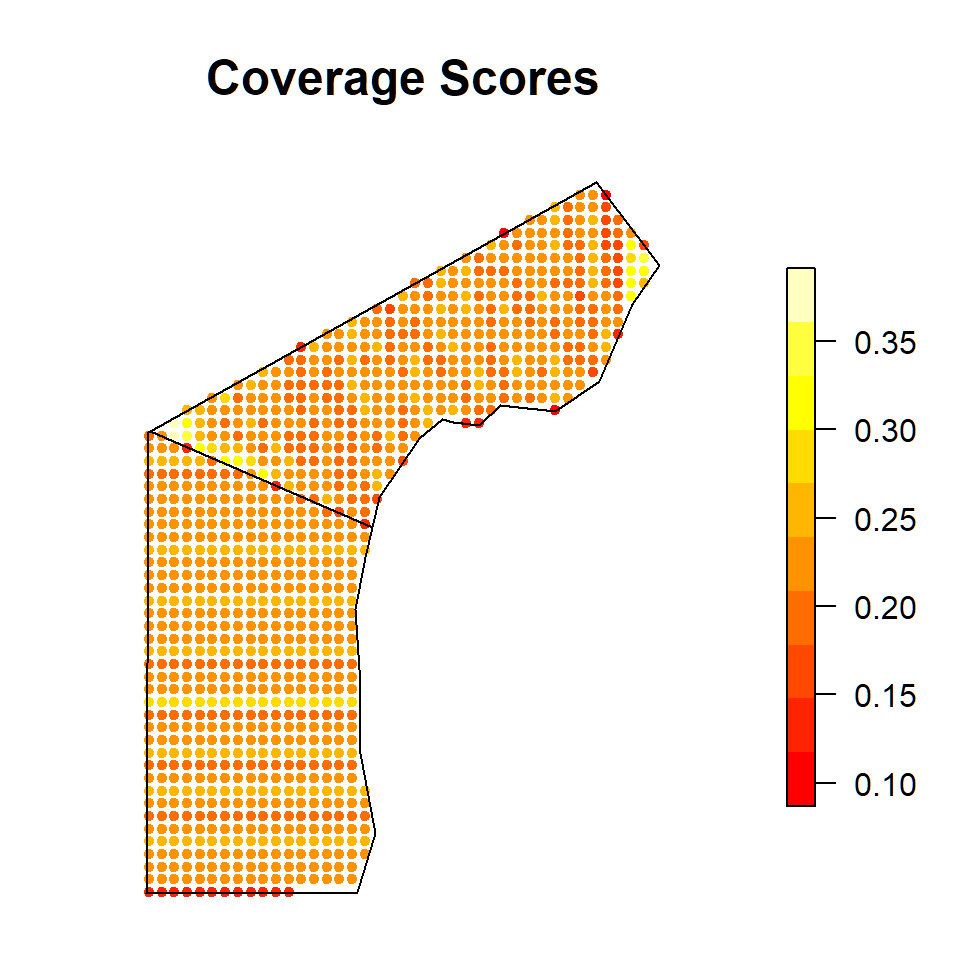Figure 6: Plot of the coverage scores for the mixed type design, based on the generation of 500 surveys from this design.

We can also look at the design statistics from the 1000 surveys generated, displayed below. We can see in this multiple strata example we are now given stratum-specific statistics as well as values for the study region as a whole in the Total columns. We can see from the strata areas information that the southern strata is slightly bigger than the northern strata and also looking at the covered area table we see that the covered area is larger in the southern strata. If we look at the % of region covered statistics we see that the coverage is approximately the same in both strata, having a percentage covered of 22.09% in the northern stratum and 22.67% in the southern stratum.


design

Strata North:
______________
Design:  equal spaced zigzag
Spacing:  NA
Number of samplers:  NA
Line length:  1200000  (shared across strata)
Design angle:  65
Edge protocol:  minus

Strata South:
______________
Design:  systematically spaced transects
Spacing:  NA
Number of samplers:  NA
Line length:  1200000  (shared across strata)
Design angle:  90
Edge protocol:  minus

Strata areas:  9280648398, 11716012130
Region and effort units:  m
Coverage Simulation repetitions:  1000

Number of samplers:

North South Total
Minimum   9.0   9.0  18.0
Mean     10.7   9.5  20.2
Median   11.0  10.0  20.0
Maximum  12.0  10.0  22.0
sd        0.6   0.5   0.8

Covered area:

North      South      Total
Minimum 1955116049 2513421503 4481285619
Mean    2049652296 2656340209 4705992505
Median  2070366837 2670394472 4713693428
Maximum 2091766860 2782781204 4870589559
sd        36486449   82822551   91678794

% of region covered:

North South Total
Minimum 21.07 21.45 21.34
Mean    22.09 22.67 22.41
Median  22.31 22.79 22.45
Maximum 22.54 23.75 23.20
sd       0.39  0.71  0.44

Line length:

North     South      Total
Minimum 497407.9 631156.05 1128751.83
Mean    521716.3 669307.22 1191023.50
Median  525731.8 670981.00 1192041.07
Maximum 535469.7 706157.31 1239785.11
sd        9013.4  23011.45   24965.31

Trackline length:

North     South      Total
Minimum 512691.24 798122.48 1311004.79
Mean    538759.40 846771.69 1385531.09
Median  541724.36 856097.28 1392394.59
Maximum 550794.04 891391.01 1440740.61
sd        8958.67  31339.93   32758.02

Cyclic trackline length:

North      South      Total
Minimum 683046.12  938100.75 1621340.14
Mean    722453.73 1005787.30 1728241.03
Median  727656.40 1031911.48 1741506.91
Maximum 737260.78 1066109.12 1801958.77
sd       13922.31   47925.48   50049.89

Coverage Score Summary:

North      South      Total
Minimum 0.0870000 0.12000000 0.08700000
Mean    0.2201493 0.22586538 0.22337377
Median  0.2190000 0.22300000 0.22200000
Maximum 0.3910000 0.27000000 0.39100000
sd      0.0309947 0.02553877 0.02817631

### Segmented Line Design

This example uses a segmented grid design to further demonstrate how design parameters can be varied across strata. In this example the spacing, segment length and segment threshold values have been specified explicitly for each strata. In the northern stratum we have segments of 5km in length separated by 10km. The segment threshold of 50 means that any segments less than 50% of the segment length (i.e. 2.5km) will be discarded. In the southern stratum we have segments of 12km separated by 20km and where we retain all segments no matter how short they are (segment threshold of 0). An example survey from this design is shown in Figure 7.


design <- make.design(region = region,
transect.type = "line",
design = "segmentedgrid",
spacing = c(10000,20000),
seg.length = c(5000,12000),
design.angle = c(150, 90),
seg.threshold = c(50,0),
edge.protocol = "minus",
truncation = 3000,
coverage.grid = cover)
transects <- generate.transects(design)
plot(region, transects, lwd = 1.5, col = 4)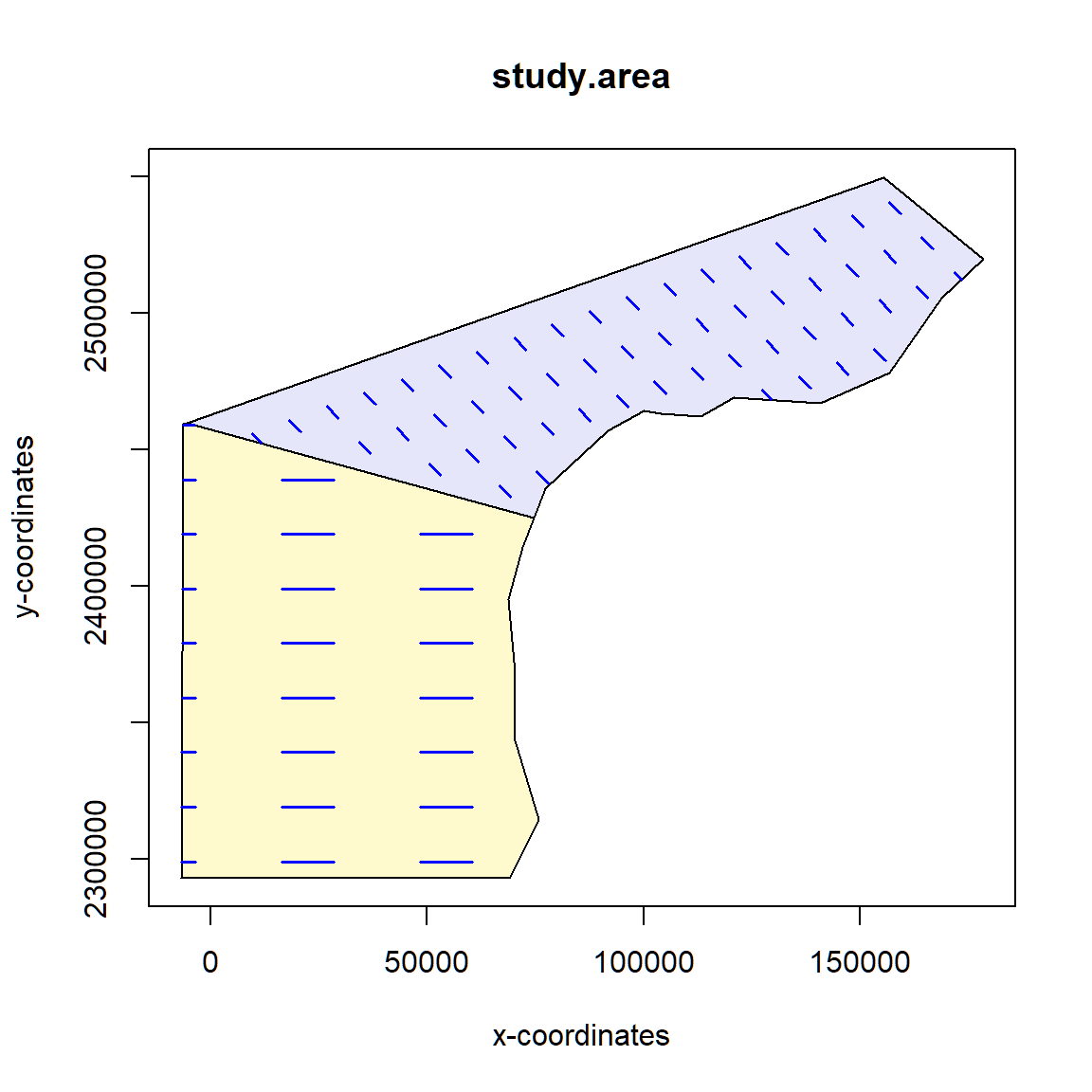Figure 7: A single survey generated from a segmented grid design with different design parameters in each stratum.

We can then have a look at the coverage scores to examine coverage in the two strata. We observe on average the coverage is higher in the northern stratum than the southern stratum because spacing was half as large in the northern stratum, Figure 8.


# You can re-run the coverage simulation using the following code. Note, your
# results should vary slightly from mine, make sure you haven't set a seed!
design <- run.coverage(design, reps = 1000)
plot(design)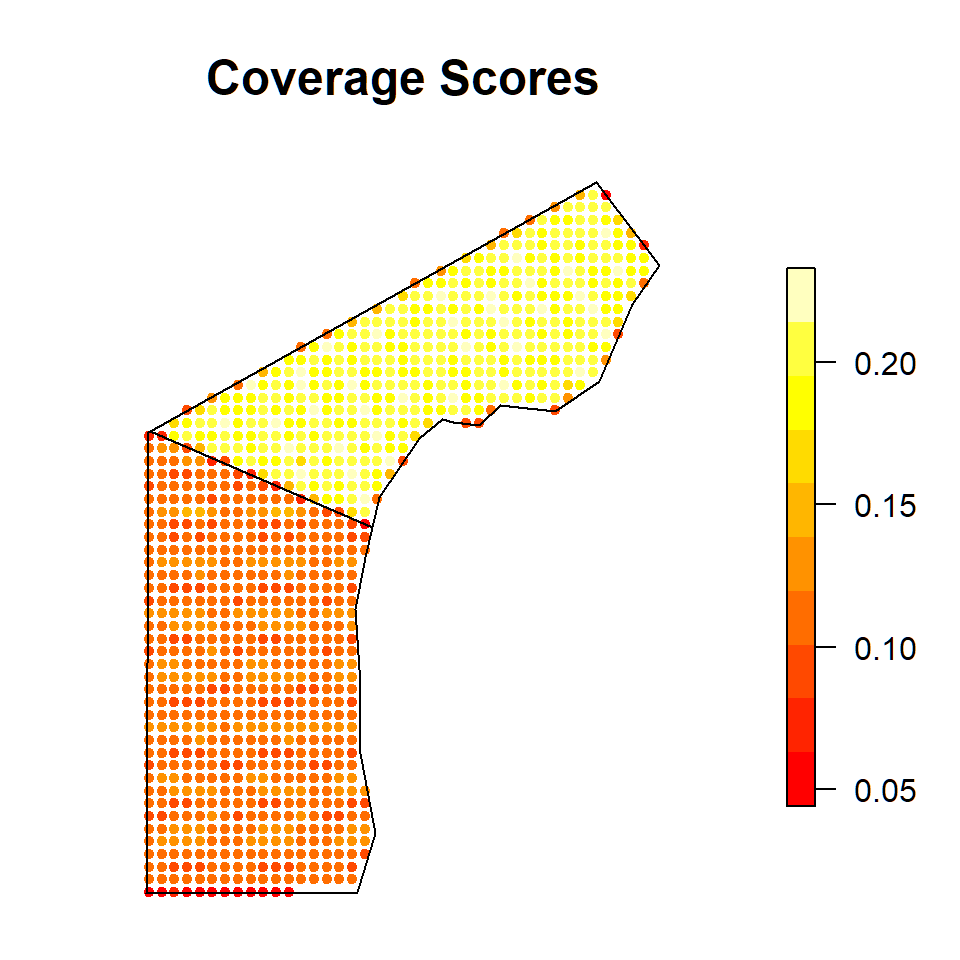Figure 8: Coverage scores plotted for the segmented grid design.

When there are differences in average coverage between strata it is sometimes difficult to visualise non-uniform coverage within strata. The survey design package also provides the functionality to view the coverage score plot for individual strata, Figures 9 and 10. While the minor edge effects in the northern stratum were apparent when the study area was plotted as a whole, Figure 8, the edge effects in the southern stratum were not detectable in this plot. However, we can see from Figure 10 that the edge effects in the southern stratum are also very small in comparison to the stratum area.


plot(design, strata.id = 1, subtitle = "Coverage Northern Strata")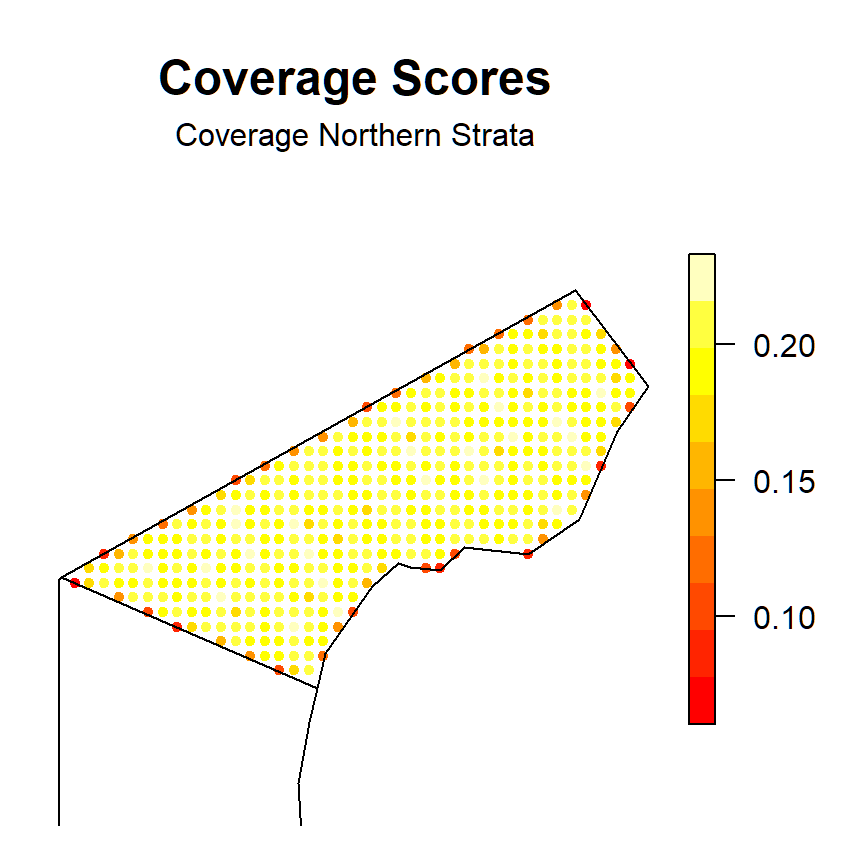Figure 9: Coverage scores plotted only for the northern stratum.


plot(design, strata.id = 2, subtitle = "Coverage Southern Strata")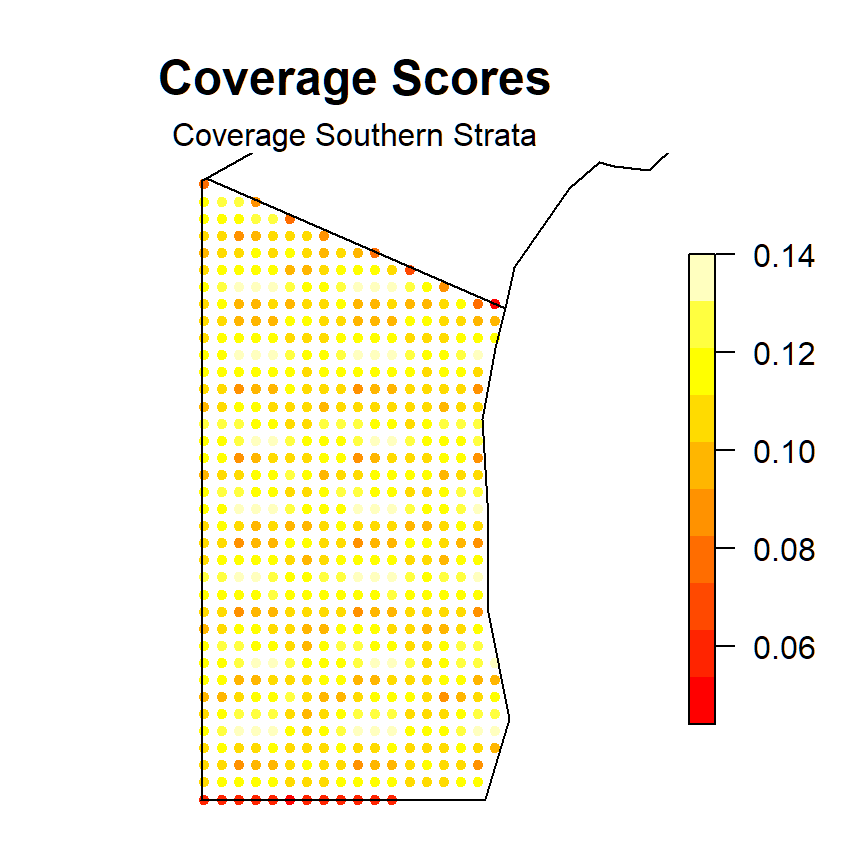Figure 10: Coverage scores plotted only for the southern stratum.

### Point transect design

For this point transect example we will use a different study region. This is an area of forest in Scotland between Dundee and St Andrews made up of two strata, Figure 11. As this region is not projected we will first need to project the study area onto a flat plane.


library(sf)
shapefile.name <- system.file("extdata", "TentsmuirUnproj.shp", package = "dssd")
# Define a European Albers Equal Area projection
proj4string <- "+proj=aea +lat_1=56 +lat_2=62 +lat_0=50 +lon_0=-3 +x_0=0
+y_0=0 +ellps=intl +units=m"
# Project the study area on to a flat plane
projected.shape <- st_transform(sf.shape, crs = proj4string)
# Create the survey region in dssd
region.tm <- make.region(region.name = "Tentsmuir",
strata.name = c("Main Area", "Morton Lochs"),
shape = projected.shape)
# Plot the survey region
plot(region.tm, legend.params = list(inset = c(-0.3,0)))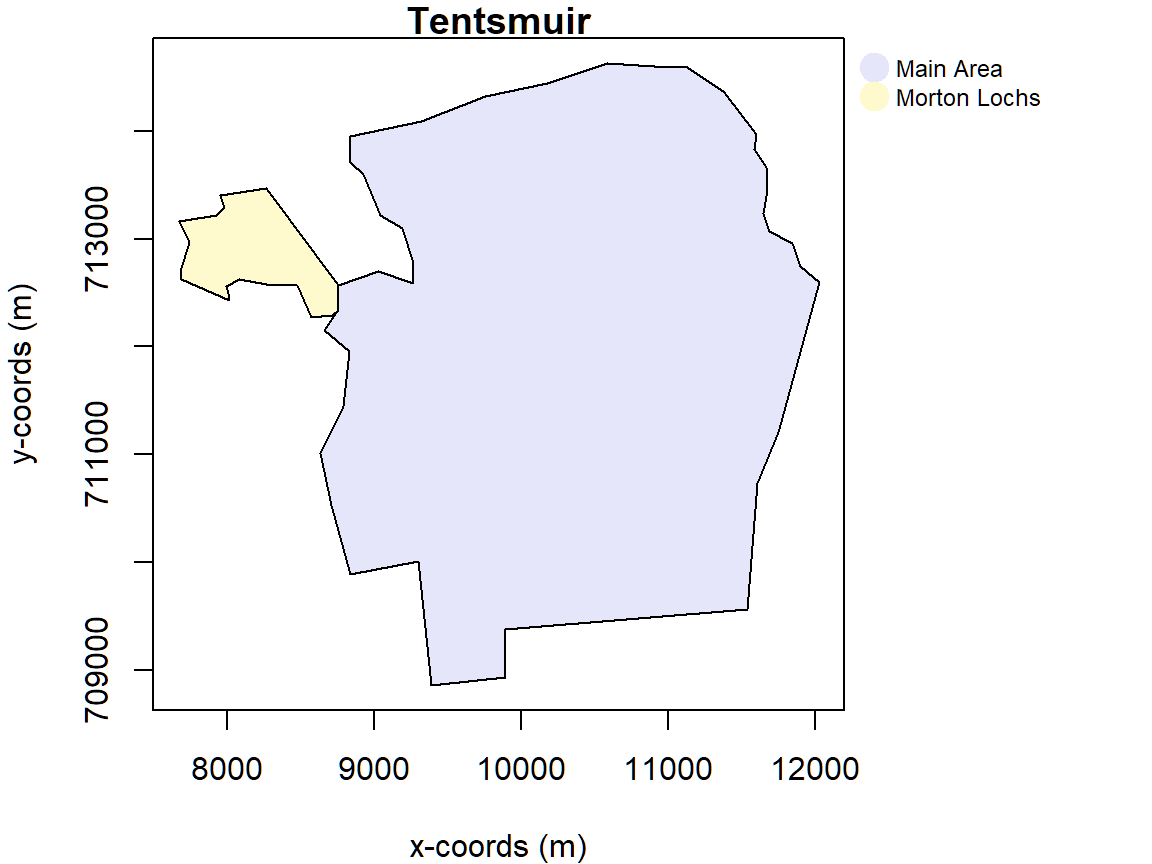Figure 11: Study region depicting an area of forest between Dundee and St Andrews in Scotland. It comprises of a main stratum and a Morton Loch stratum which forms part of a nature reserve in the area.


#Create a coverage grid
cover.tm <- make.coverage(region.tm, n.grid.points = 500)

Point transect designs and point transect design parameters can be varied across strata in the same way as line transect designs. Here we use a systematic grid of point in both the main and Morton Lochs strata. We specify that there should be approximately 80 samplers across both strata and that we would like 25% of the effort allocated to the Morton Lochs strata and 75% to the main strata. As the Morton Lochs strata is such a small area it could be prone to edge effects so we will use a plus sampling strategy. Figure 12 shows one possible realisation of this design.


design.tm <- make.design(region = region.tm,
transect.type = "point",
design = "systematic",
samplers = 80,
effort.allocation = c(0.75,0.25),
edge.protocol = c("minus","plus"),
truncation = 100,
coverage.grid = cover.tm)
transects.tm <- generate.transects(design.tm)
plot(region.tm, transects.tm, lwd = 1.5, col = 4)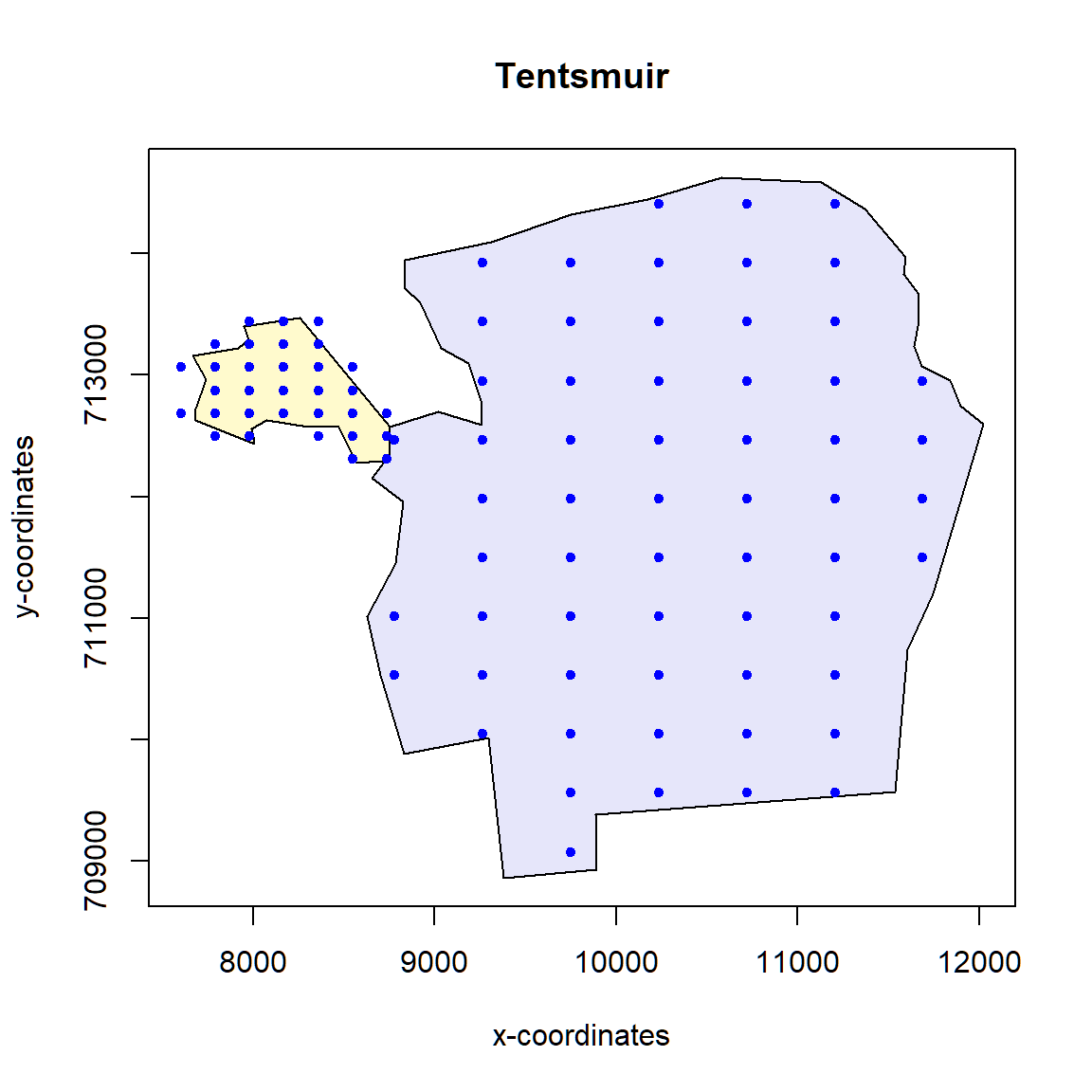Figure 12: An example multi strata point transect survey.

We can also have a look at the statistics for this particular survey. The output below shows that although we set the effort to be 80 samplers in total we have 92. While we see the expected number in the main stratum 75% of 80 is 60 samplers, we see more than expected in the Morton Lochs stratum, 32 rather than 20. This is down to the fact that we are using a plus sampling edge protocol in this stratum and if we check in Figure 12 above we will see that approximately 20 points actually fall inside the Morton Lochs stratum. This additional effort required for the plus sampling strategy is to be taken into account when costing for your survey.


transects.tm

Strata Main Area:
__________________
Design:  systematically spaced transects
Spacing:  484.9165
Number of samplers:  60
Design angle:  0
Edge protocol:  minus
Covered area:  1842894
Strata coverage: 13.06%
Strata area:  14108643

Strata Morton Lochs:
_____________________
Design:  systematically spaced transects
Spacing:  189.1117
Number of samplers:  32
Design angle:  0
Edge protocol:  plus
Covered area:  630454.5
Strata coverage: 88.14%
Strata area:  715264.9

Study Area Totals:
_________________
Number of samplers:  92
Effort allocation: 75%, 25%
Covered area:  2473348
Average coverage: 16.68%

### Manual creation of multiple strata study areas

Example of creating a study region manually. This example code is taken from the help file for ‘sf::st_multipolygon’. To create an sf polygon or multipolygon, create one or more matrices of coordinates representing the outer polygons and any holes. For multipolygons the outer polygons and holes are defined by their placement within their list element, only the first matrix in a list element is an outer polygon then all the following matrices are holes. In the following example all polygons would be considered to be in the same strata.


outer <- matrix(c(0,0,15,0,15,10,0,10,0,0),ncol=2, byrow=TRUE)
hole1 <- matrix(c(2,2,2,3,3,3,3,2,2,2),ncol=2, byrow=TRUE)
hole2 <- matrix(c(5,5,5,6,7,6,8,5.5,7,5,5,5),ncol=2, byrow=TRUE)
pol1 <- list(outer, hole1*1.5, hole2)
pol2 <- list(outer + 15, hole2*1.5 + 12)
pol3 <- list(outer + 30, hole2*2.5 + 20)
mp <- list(pol1,pol2,pol3)
mp1 <- sf::st_multipolygon(mp)

region <- make.region(region.name = "study.area", shape = mp1)
plot(region)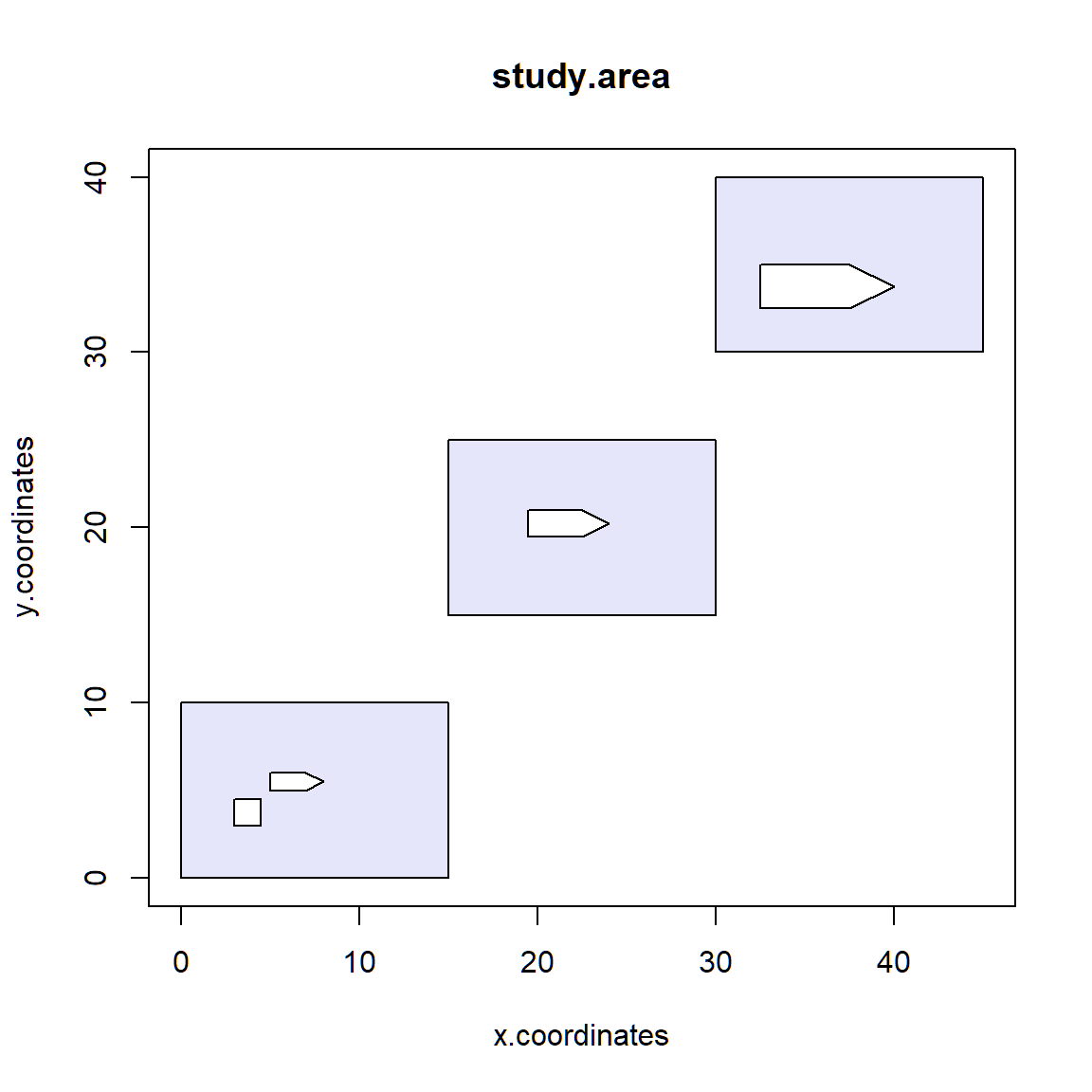Figure 13: A single study region with multiple polygon parts.

If instead you wanted to create the 3 separate polygons as 3 distinct strata you could use the following code:


outer <- matrix(c(0,0,15,0,15,10,0,10,0,0),ncol=2, byrow=TRUE)
hole1 <- matrix(c(2,2,2,3,3,3,3,2,2,2),ncol=2, byrow=TRUE)
hole2 <- matrix(c(5,5,5,6,7,6,8,5.5,7,5,5,5),ncol=2, byrow=TRUE)
pol1 <- sf::st_polygon(list(outer, hole1*1.5, hole2))
pol2 <- sf::st_polygon(list(outer + 15, hole2*1.5 + 12))
pol3 <- sf::st_polygon(list(outer + 30, hole2*2.5 + 20))
sfc <- sf::st_sfc(pol1,pol2,pol3)
strata.names <- c("SW", "central", "NE")
mp1 <- sf::st_sf(strata = strata.names, geom = sfc)

region <- make.region(region.name = "study.area",
strata.name = strata.names,
shape = mp1)
plot(region)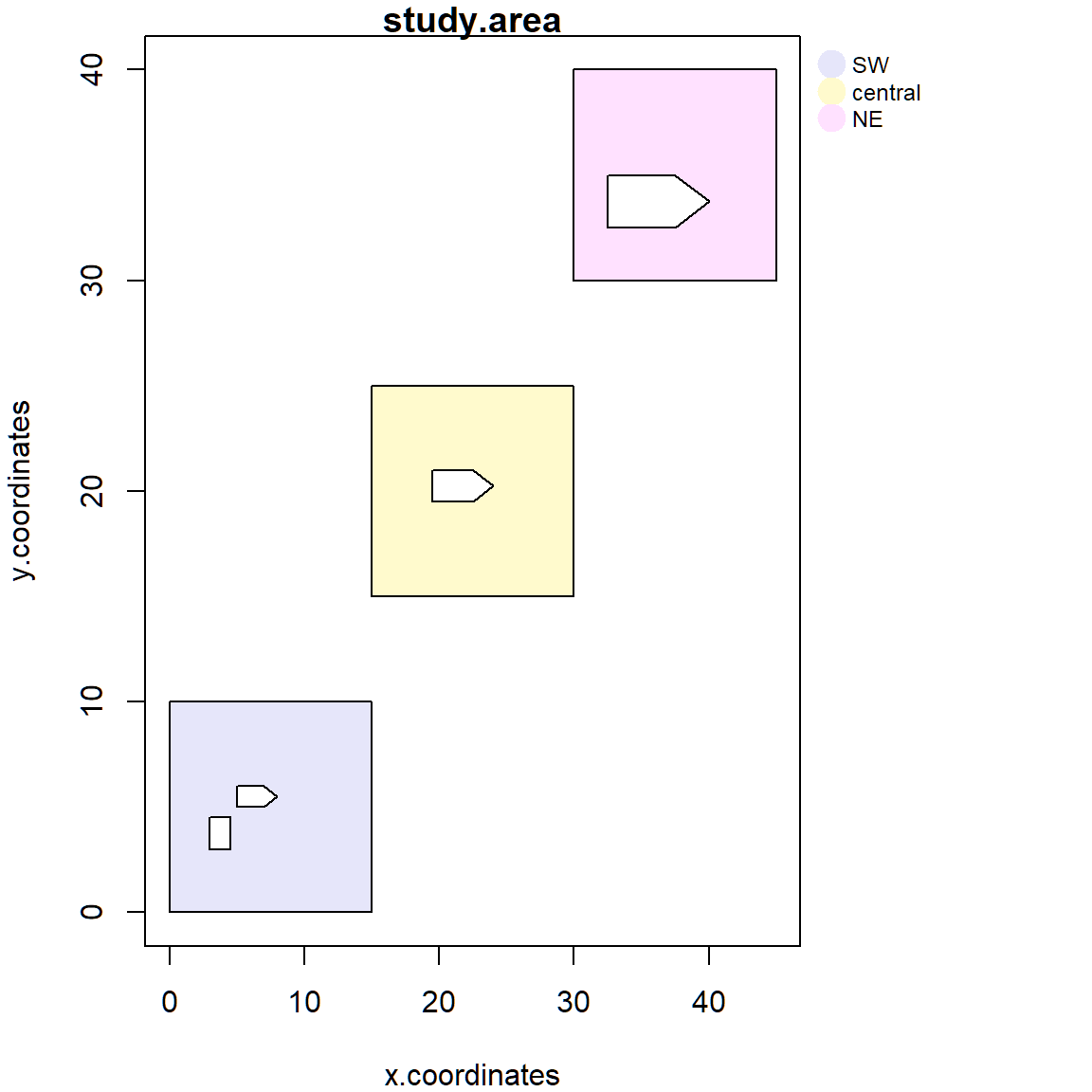Figure 14: A single study region with multiple polygon parts. Each are separate strata.